Next: Determination of Phase-Shifts Up: Scattering Theory Previous: Born Approximation

# Partial Waves

We can assume, without loss of generality, that the incident wavefunction is characterized by a wavevectorwhich is aligned parallel to the-axis. The scattered wavefunction is characterized by a wavevector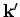which has the same magnitude as, but, in general, points in a different direction. The direction ofis specified by the polar angle(i.e., the angle subtended between the two wavevectors), and an azimuthal angle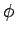about the-axis. Equations (1269) and (1270) strongly suggest that for a spherically symmetric scattering potential [i.e.,] the scattering amplitude is a function ofonly: i.e.,(1282)

It follows that neither the incident wavefunction,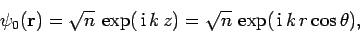(1283)

nor the large-form of the total wavefunction,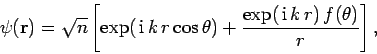(1284)

depend on the azimuthal angle.

Outside the range of the scattering potential, both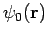andsatisfy the free space Schrödinger equation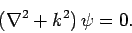(1285)

What is the most general solution to this equation in spherical polar coordinates which does not depend on the azimuthal angle? Separation of variables yields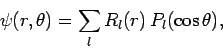(1286)

since the Legendre functions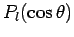form a complete set in-space. The Legendre functions are related to the spherical harmonics, introduced in Cha. 8, via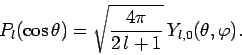(1287)

Equations (1285) and (1286) can be combined to give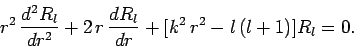(1288)

The two independent solutions to this equation are the spherical Bessel functions,and, introduced in Sect. 9.3. Recall that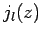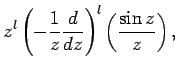(1289)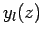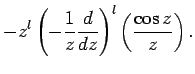(1290)

Note that theare well-behaved in the limit, whereas thebecome singular. The asymptotic behaviour of these functions in the limit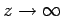is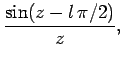(1291)(1292)

We can write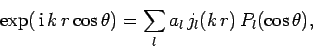(1293)

where the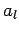are constants. Note there are nofunctions in this expression, because they are not well-behaved as. The Legendre functions are orthonormal,(1294)

so we can invert the above expansion to give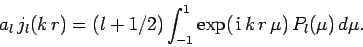(1295)

It is well-known that(1296)

where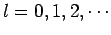[see M. Abramowitz and I.A. Stegun, Handbook of mathematical functions, (Dover, New York NY, 1965), Eq. 10.1.14]. Thus,(1297)

giving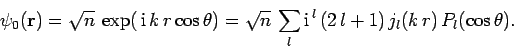(1298)

The above expression tells us how to decompose the incident plane-wave into a series of spherical waves. These waves are usually termed partial waves''.

The most general expression for the total wavefunction outside the scattering region is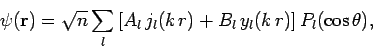(1299)

where theandare constants. Note that thefunctions are allowed to appear in this expansion, because its region of validity does not include the origin. In the large-limit, the total wavefunction reduces to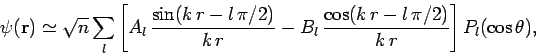(1300)

where use has been made of Eqs. (1291) and (1292). The above expression can also be written(1301)

where the sine and cosine functions have been combined to give a sine function which is phase-shifted by. Note that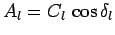and.

Equation (1301) yields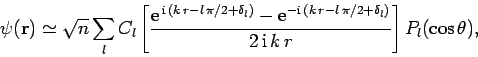(1302)

which contains both incoming and outgoing spherical waves. What is the source of the incoming waves? Obviously, they must be part of the large-asymptotic expansion of the incident wavefunction. In fact, it is easily seen from Eqs. (1291) and (1298) that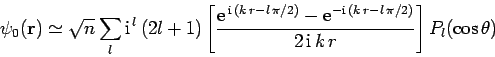(1303)

in the large-limit. Now, Eqs. (1283) and (1284) give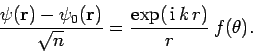(1304)

Note that the right-hand side consists of an outgoing spherical wave only. This implies that the coefficients of the incoming spherical waves in the large-expansions ofandmust be the same. It follows from Eqs. (1302) and (1303) that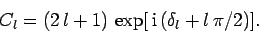(1305)

Thus, Eqs. (1302)-(1304) yield(1306)

Clearly, determining the scattering amplitude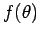via a decomposition into partial waves (i.e., spherical waves) is equivalent to determining the phase-shifts.

Now, the differential scattering cross-section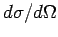is simply the modulus squared of the scattering amplitude[see Eq. (1266)]. The total cross-section is thus given by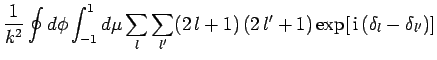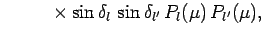(1307)

where. It follows that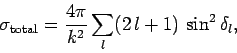(1308)

where use has been made of Eq. (1294).Next: Determination of Phase-Shifts Up: Scattering Theory Previous: Born Approximation
Richard Fitzpatrick 2010-07-20